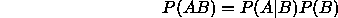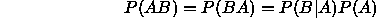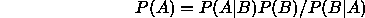Next: Maximum Likelihood Up: Statistical Background Previous: Basic Definitions of

## Bayes Theorem

The basic foundation of probability theory follows from the following intuitive definition of conditional probability.In this definition events A and B are simultaneous an have no (explicit) temporal order we can writeThis leads us to a common form of Bayes Theory, the equation:which allows us to compute the probability of one event in terms of observations of another and knowledge of joint distributions.

Bob Fisher
Fri Mar 28 14:12:50 GMT 1997